Ex 13.8

Chapter 13 Class 9 Surface Areas and Volumes
Serial order wise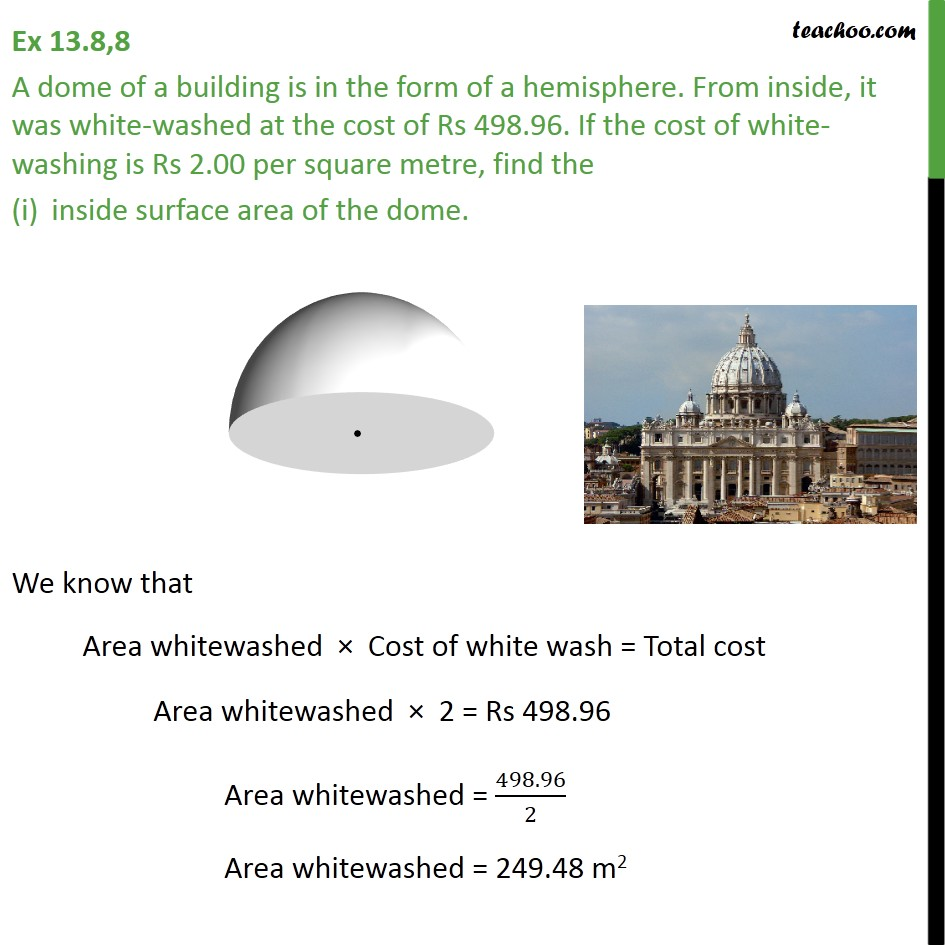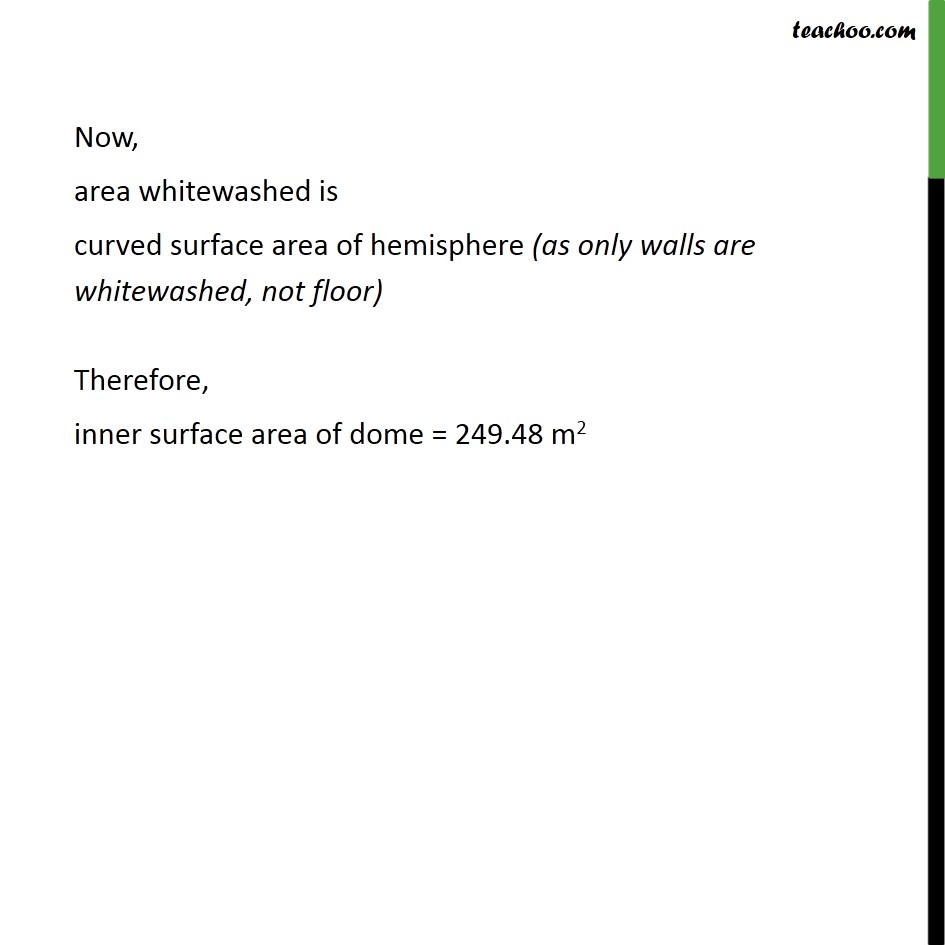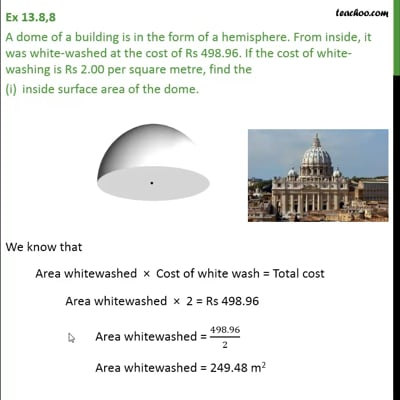This video is only available for Teachoo black users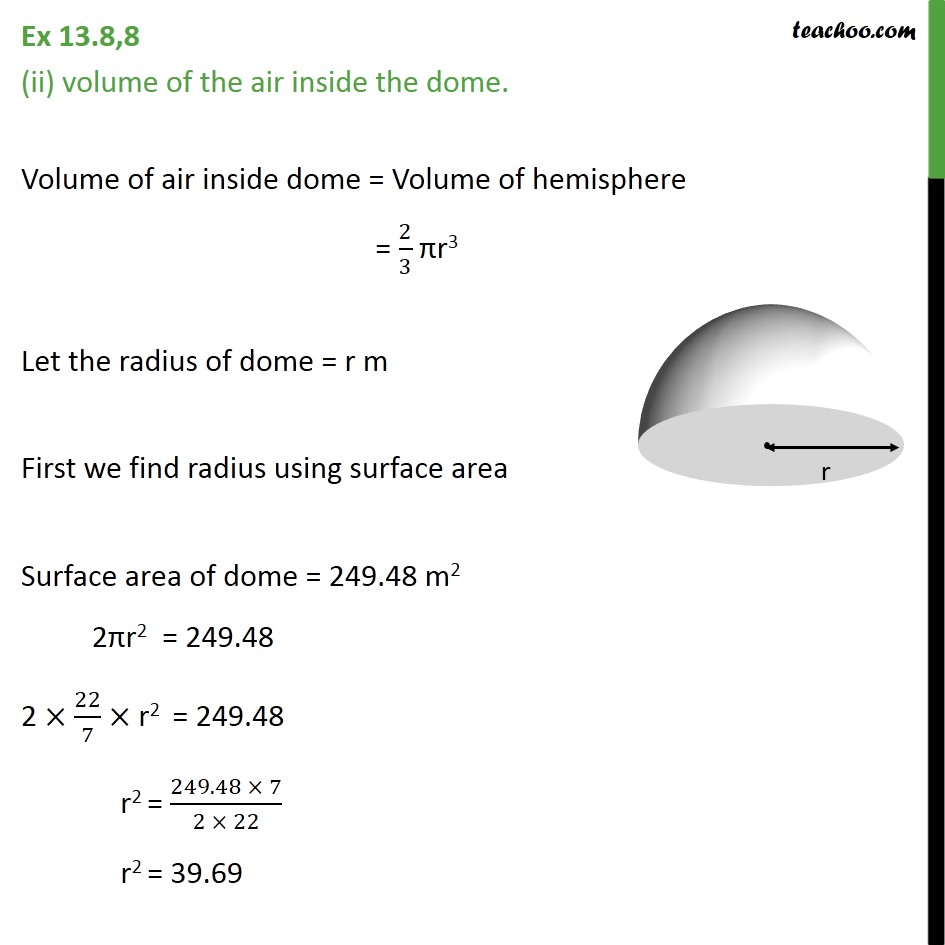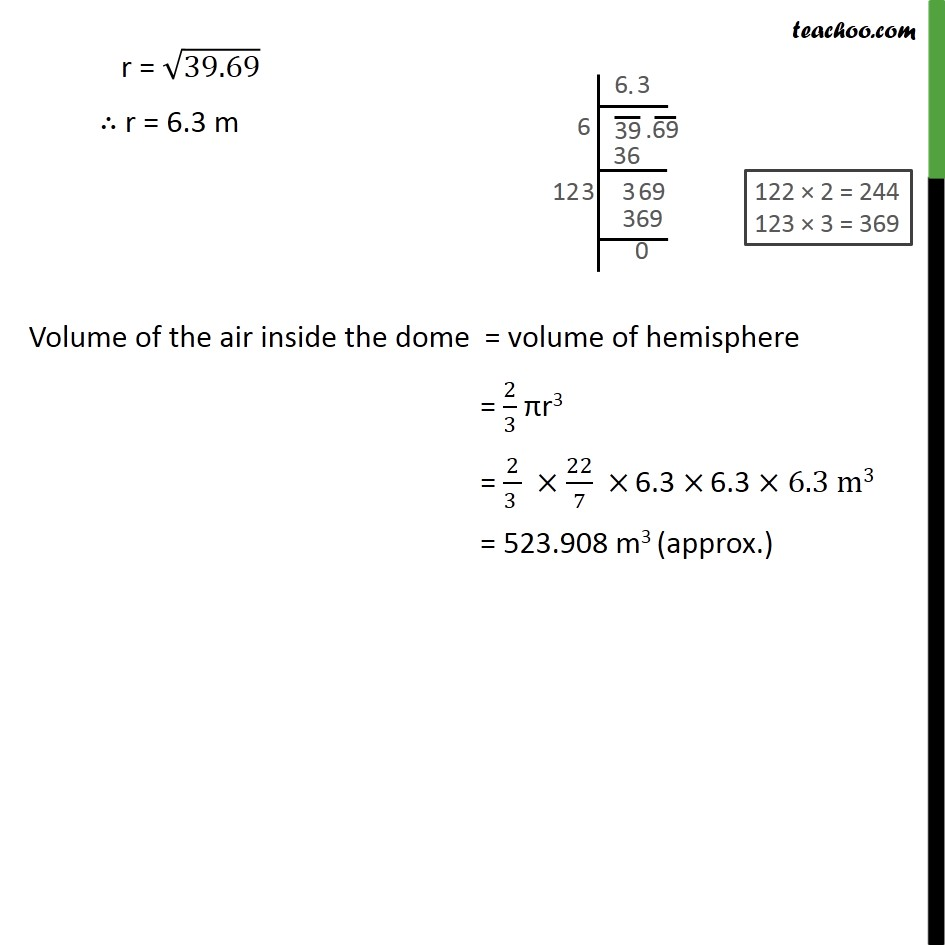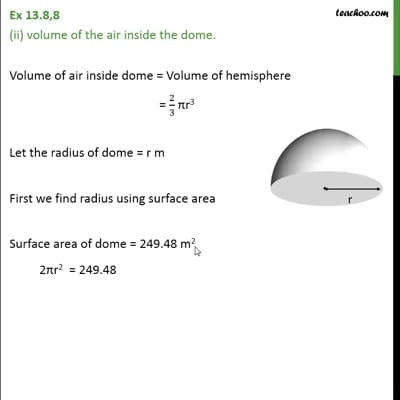This video is only available for Teachoo black users

Maths Crash Course - Live lectures + all videos + Real time Doubt solving!

### Transcript

Ex 13.8,8 A dome of a building is in the form of a hemisphere. From inside, it was white-washed at the cost of Rs 498.96. If the cost of white-washing is Rs 2.00 per square metre, find the inside surface area of the dome. We know that Area whitewashed × Cost of white wash = Total cost Area whitewashed × 2 = Rs 498.96 Area whitewashed = 498.96/2 Area whitewashed = 249.48 m2 Now, area whitewashed is curved surface area of hemisphere (as only walls are whitewashed, not floor) Therefore, inner surface area of dome = 249.48 m2 Ex 13.8,8 (ii) volume of the air inside the dome. Volume of air inside dome = Volume of hemisphere = 2/3 πr3 Let the radius of dome = r m First we find radius using surface area Surface area of dome = 249.48 m2 2πr2 = 249.48 2 × 22/7 × r2 = 249.48 r2 = (249.48 × 7)/(2 × 22) r2 = 39.69 r = √39.69 ∴ r = 6.3 m Volume of the air inside the dome = volume of hemisphere = 2/3 πr3 = 2/(3 ) ×22/7 × 6.3 × 6.3 ×6.3 m3 = 523.908 m3 (approx.)